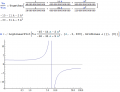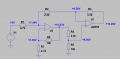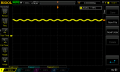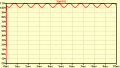# Cascaded Op Amp circuit .. #2

#### The Electrician

Joined Oct 9, 2007
2,866
I agree, of course.
But the question is not how an ideal opamp is to be defined, but:
Does it matter if we consider an opamp as ideal or real - as far as the stability of the DC operating point is concerned
This involves the loop gain only (and the stability criterion). In both cases, the loop gain > +1.
So the circuit will not assume a stable DC operating point.

This will not stabilize the circuit.
Suppose the opamps have a gain of zero. Then the outputs of the opamps will be essentially a short to ground. The circuit can then be analyzed as a purely passive network of resistors. Now imagine that the gain of the opamps is very small, perhaps 1/10. WIll the circuit latch up then? My analysis indicates that for sufficiently small opamp gains the circuit will be stable.

What do you calculate as the loop gain when the opamps have gains of .1 or 1?

#### LvW

Joined Jun 13, 2013
1,337
Suppose the opamps have a gain of zero. Then the outputs of the opamps will be essentially a short to ground. The circuit can then be analyzed as a purely passive network of resistors. Now imagine that the gain of the opamps is very small, perhaps 1/10. WIll the circuit latch up then? My analysis indicates that for sufficiently small opamp gains the circuit will be stable.

What do you calculate as the loop gain when the opamps have gains of .1 or 1?
Of course, I can calculate the loop gain for the circuit assuming a DC open-loop gain of "zero" (do you realy mean zero?) or 0.1.
A nice mathematical exercise - but for which purpose? (Or were you joking with me?)

#### ericgibbs

Joined Jan 29, 2010
14,190

#### LvW

Joined Jun 13, 2013
1,337
hi 442,
This is what LTSpice shows...
@arthur442
E
Hi Eric,
I will comment your result. It is a typical example for the case, where the simulator is correct - however, the interpretation from the user is not.
Let me use a short mechanical example:
A small ball is riding upon a bigger one. In theory, this could be possible assuming that there is absolutely no external perturbation (mechanical, by air,...). This is completely unrealistic, so the system is unstable under all real-world conditions.

In our example, we face the same situation:
From the idealized mathematical point of view the results in your simulation are correct - because, in your analysis you have assumed that
(a) the power supplies are switched on since infinite times already (no switch-on effect) and
(b) are absolutely fixed (no variations)
(c) that the opamps are ideal (without any delay) and
(d) there are no external influences like noise....

Everything is idealized as in the mechanical example.

Make a new test:
* Use real opamp models,
* switch on the supply voltage at t=0
* or use a small varying voltage (sin source) in series to the DC input .

As a result, you will see that the circuit will go immediately into saturation (no surprise because of postive feedback >1)

A very simple, but similar case:
Use one single opamp (real or ideal) and provide it with a postive feedback.
Any simulation under idealized conditions (as mentioned above) cannot reveal any instabilities. This is something each user should know.

Last edited:

#### The Electrician

Joined Oct 9, 2007
2,866
Of course, I can calculate the loop gain for the circuit assuming a DC open-loop gain of "zero" (do you realy mean zero?) or 0.1.
A nice mathematical exercise - but for which purpose? (Or were you joking with me?)
I'm speaking of the opamp gains. The question here is stability. I believe that if the gains of the opamps (I'm assuming that the opamps are identical; they don't have to be however) are low enough the circuit will be DC stable. If the gains are .1, what do you calculate for the loop gain? Will that be stable?

If the feedback path has attenuation, won't the opamps require more gain that in order for latchup to occur?

Last edited:

#### The Electrician

Joined Oct 9, 2007
2,866

LvW said "A very simple, but similar case:
Use one single opamp (real or ideal) and provide it with a postive feedback.
Any simulation under idealized conditions (as mentioned above) cannot reveal any instabilities. This is something each user should know. "

Here's an example of this:

Go down to the heading "Closed-loop amplifier". There is a schematic of a simple non-inverting amplifier, and there is an analysis to determine the closed-loop gain.#### LvW

Joined Jun 13, 2013
1,337
I'm speaking of the opamp gains. The question here is stability. I believe that if the gains of the opamps (I'm assuming that the opamps are identical; they don't have to be however) are low enough the circuit will be DC stable. If the gains are .1, what do you calculate for the loop gain? Will that be stable?
Why are you asking a question which has no relation to the original described problem?
Of course, any instable system can be stabilized by changing gain values correspondingly - no surprise at all.
In this specific case, the loop gain for DC will be smaller than unity, but still positive. Simple calculation.
I am sure you know what this means - why are you asking?

#### LvW

Joined Jun 13, 2013
1,337

LvW said "A very simple, but similar case:
Use one single opamp (real or ideal) and provide it with a postive feedback.
Any simulation under idealized conditions (as mentioned above) cannot reveal any instabilities. This is something each user should know. "

Here's an example of this:

Go down to the heading "Closed-loop amplifier". There is a schematic of a simple non-inverting amplifier, and there is an analysis to determine the closed-loop gain.

View attachment 243540
Just one comment.
Of course, no surprise.
Writing down the first line in your calculations, you already assume that it is an amplifier that can work within a linear region.
But this assumption does not apply to an instable system.
Hence, you must always know in advance if the sysytem under consideration is stable or not.
Otherwise, you have to (as a first step) a stability analysis. Therefore, I have calculated the loop gain for the given circuit and values.
This is the classical method for anylyzing (unknown) sysytems with feedback.

Background: Instability is DEFINED in relation to an answer to the following question:
How will the bias point (within a quasi-linear region of the transfer function) react upon a small perturbation within the system.

#### The Electrician

Joined Oct 9, 2007
2,866
Just one comment.
Of course, no surprise.
Writing down the first line in your calculations, you already assume that it is an amplifier that can work within a linear region.
But this assumption does not apply to an instable system.
Hence, you must always know in advance if the sysytem under consideration is stable or not.
Otherwise, you have to (as a first step) a stability analysis. Therefore, I have calculated the loop gain for the given circuit and values.
This is the classical method for anylyzing (unknown) sysytems with feedback.

Background: Instability is DEFINED in relation to an answer to the following question:
How will the bias point (within a quasi-linear region of the transfer function) react upon a small perturbation within the system.
As you and I have discussed in another post, the classical method can be difficult to perform when there is more than one feedback path. A method that always works is to use information available with nodal analysis.

ericgibbs, can you do simulations with opamp gains of 5 and 10, and follow LvW's suggestion of adding some kind of perturbation at t=0?

#### The Electrician

Joined Oct 9, 2007
2,866
A technique that can be used to determine if an opamp circuit like this is unstable is to calculate the transfer function from the input to other nodes, then multiply by the input voltage. Plot node voltage(s) variation as the opamp gain A varies from zero to some high value and see if that voltage exhibits a singularity. Here is that plot of the voltage at Va as A varies.

In the plot, the vertical axis is the voltage at Va and the horizontal axis is the value of A. As can be seen, the voltage at Va goes to infinity when A is about 7.051; This means that the circuit will latch up when A is larger than the singularity value.However, if the + and - inputs of the rightmost opamp are reversed, the plot of Va looks like this:The circuit is DC stable now.

#### LvW

Joined Jun 13, 2013
1,337
ericgibbs, can you do simulations with opamp gains of 5 and 10, and follow LvW's suggestion of adding some kind of perturbation at t=0?
I have done such simulations (PSpice) for the circuit (and values) as given in the 1st post (however, with dual power supply).
These results were the basis for the contents of my post#32

Last edited:

#### LvW

Joined Jun 13, 2013
1,337
Hi Eric, may I direct your attention to the following:
1.) An ac analysis starts with finding the DC bias point of the circuit - without any power supply switch-on transient.
As seen (and mentioned) before the circuit under investigation has no stable bias point - under realsitic conditions.
Therefore, a closed-loop ac analysis can NEVER reveal such DC instabilities.
The same applies to a pure DC analysis.

2.) There are only two safe and correct methods for investigating the stability properties (AC and DC, resp.) of a circuit with feedback:
* Open-loop ac analysis (loop gain) with application of the well-known stability criterion (provided the DC bias point is correct):
* Tran analysis for the closed-loop system with real opamp models - and power switch-on at t=0.

3.) Comment: Why do you think that "at higher frequencies" the circuit shows instability? The phase response shows a surprising increase - but how and why is this an indication for instability ? Which criterion for stability did you apply?

4.) Instead, a simple visual inspection of the circuit - together with the shown DC values - reveals that "something" is wrong with your bias point:
There are no phase inversions within the circuit ....all inputs are connected to the non-inverting opamp terminals.
Nevertheless, the output is negative for a positive input - this is impossible for a circuit working in the linear input-output transfer region.

Last edited:

#### Jony130

Joined Feb 17, 2009
5,252
I decided to test this circuit on the breadboard using LM358 and dual supply +/-12V

I use this schematic (DC voltage measured in a real circuit )And the output voltage on the scope for Vin = 5VDC + 1Vp (1Khz)The similar things we can see on LTspiceHowever, if the + and - inputs of the rightmost opamp are reversed, the plot of Va looks like this:
The circuit is DC stable now.
Maybe it is a DC stable now. But on the bench, after swapping the inputs circuit start to oscillate (Fosc = 14Khz).
As we can see on the scope:•ericgibbs

#### LvW

Joined Jun 13, 2013
1,337
Hi Jony - no surprise.
A simple visual inspection shows that - after swappig the input nodes of the 2nd opamp - it will have a positive DC feedback loop.
It is really a weird circuit - and, actually, it does not deserve so many comments (in total 45).

#### MrAl

Joined Jun 17, 2014
8,556
Hello,

I found this to be an interesting circuit and the discussion that followed was also interesting.
I did not see Jony's nice schematic so i cleaned up the first image in this thread but i labeled all the resistors the same as his schematic so there would be no difference in the part numbering, and also added letters for all the nodes A through G with G being ground.

A couple things jumped out at me right off.

The first was that i dont think this is a true cascade circuit because the second stage influences the first stage and i dont think we see that in cascade circuits, so this circuit seems like just one circuit to me.

The second is that there is no negative supply voltage so there can not be any negative results for any outputs. This has been noted by others too.

Third, when we analyze a circuit we have to recognize the paradigm we are working under. That means we have to pay attention to the main intentions of the author as to how they want us to interpret the diagram. This usually means what kind of models are being used and if any parasitics are to be considered. Realize that if we don tdo this then we can probably state that no schematic diagram ever represents any circuit that could possibly work. Even a simple resistor we can say has temperature variation so we can never get the stated output voltage for any circuit unless the temperature variation is given as well as the nominal component values.
This is what the wonderful world of theory involves ... an interpretation of reality. How each one of us interprets the drawing relies on what theoretical paradigm we choose to work under.

With that in mind, when we see the lack of a negative supply voltage yet two very negative voltages (chuckle) we might note right away that something is not exactly as clean as we would like to see, and that means that other things may be amiss as well.

One of those other things is the positive feedback. However, not all circuits with positive feedback oscillate. In fact, some circuits are designed with intended positive feedback in order to speed up the response or increase the input impedance. There are of course limitations to these techniques, but it's enough to know that there are some circuits with positive feedback that work fine so we can not simply state that any given circuit latches up because it has positive feedback. It has to be analyzed, and analyzed very carefully.
But then back to the theoretical parasigm ... we may have to accept that the author wanted us to analyze it as if the circuit was stable all along. It may be worth noting to them of course if that communication is possible.

I will also take a careful look at this circuit and see if i can find anything else interesting. In the mean time i have the cleaned up drawing attached, a little late but it may helpNOTE: R7 is the 5k resistor and R6 is the 10k connected to that 5k resistor. The graphic has to be modified a little as the part values somehow got shifted over a little bit.

Last edited:

#### MrAl

Joined Jun 17, 2014
8,556
Hi Jony - no surprise.
A simple visual inspection shows that - after swappig the input nodes of the 2nd opamp - it will have a positive DC feedback loop.
It is really a weird circuit - and, actually, it does not deserve so many comments (in total 45).
Hi,

Maybe more by now, but these kind of circuits are what brings the finer points of analysis out. Without considering circuits like this we would never learn some concepts.
I also still cant understand why some people feel the need to try to limit other people's ideas about a topic.
PM me if you would like to discuss this.

#### The Electrician

Joined Oct 9, 2007
2,866
hi Al,
If you prefer I could create a new Thread and move the latest posts to that Thread.?

You can then continue discussing the circuit type.
E
@MrAl
Yes, please do so. I, for one would like to continue to discuss it. I have some IEEE papers dealing with the problem of determining whether a circuit even has any stable DC operating points.

•